# TensorFlow BERT & Transformer Examples

As part of the TensorFlow series, this article focuses on coding examples on BERT and Transformer. These examples are:

• IMDB files: Sentimental analysis with pre-trained TF Hub BERT model and AdamW,
• GLUE/MRPC BERT finetuning,
• Transformer for language translation.

# IMDB files: Sentimental analysis with pre-trained TF Hub BERT model and AdamW

In this example, we use a pre-trained TensorFlow Hub model for BERT and an AdamW optimizer. Because most of the heavy work is done by the TF Hub model, we will keep the explanation simple for this example.

First, we download and prepare IMDB files.

Next, we prepare the datasets.

We will create a model containing a pre-trained BERT preprocessing layer and a pre-trained BERT processing layer. Then, we add a classification head that contains a dropout and a dense layer.

Next, we instantiate a new model to be trained with an AdamW optimizer. Once it is fitted, we use it to evaluate the testing data.

Export the model

The last part of the code exports and reload the SavedModel.

# GLUE/MRPC BERT finetuning

In this example, we finetune a BERT model for the “glue/mrpc” dataset. This dataset labels whether two sentences are semantically equivalent.

In this example, we use a pre-trained model also. Its configuration, vocabulary, and the checkpoint are stored in the remote storage gs_folder_bert. Here is a directory listing for gs_folder_bert.

gs_folder_bert contains the BERT model trained in the pre-training phase in BERT. We are going to finetune a BERT classifier for MRPC.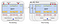Source

In line 25, we load the datasets into “glue”. It is a Python dictionary that contains the training, testing, and validation data after the loading.

Next, we will create a tokenizer that adapts to the vocabulary of the training data. We will use this tokenizer to convert a text into a sequence of integers — one integer token index for each token.

Each sample contains two sentences. We add a [CLS] token (a classification token) to indicate the start of a sample and a [SEP] token at the end of each sentence. The prepared data will feed into a BERT model all-at-once.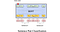Source (Our model will have an extra [SEP] at the end of sentence B)

In the method bert_encode below, it extracts sentences 1 and 2 from the dictionary glue_dict, prepares them, and encodes them into an integer sequence. Finally, we return three data structures:

• input_word_ids: the integer sequences, one integer per token,
• input_mask: indicates whether an input token contains a padded value 0 (details later), and
• input_type_ids: indicates whether the token i belongs to sentence 1 (value 0) or 2 (value 1).

Given a text input, input_type_ids is equivalent to the segment embeddings in the BERT paper. In the example below, the first six tokens belong to sentence 1 and marked as 0 in input_type_ids. The rests are 1.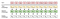Source

Next, we call bert_encode to encode the training, validation, and testing test.

We encode samples in the whole dataset all at once. Therefore, glue_train contains all 3688 samples and input_word_ids will have a shape of (3668, 103), (number of samples, sequence length).

The longest sample in the training dataset has 103 tokens. And all training samples are right padded with 0 to this longest sequence. This allows the samples to be trained as a Tensor. The padding is done by to_tensor(). Here is an example of how this method works.

The input_mask indicates whether the token i holds a padded 0 or now. A padded token should be ignored and output a default value, say 0. This mask is purposely created as a separate tensor so it can be passed to the subsequent layers. Next, we will create a BERT model. The configuration is stored in the remote directory gs_folder_bert. Here is the content of the configuration file.

Just as a reference, this is the model summary for the BERT model created (the encoder of a Transformer).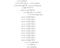Source

Then, we use the model to test out 10 training samples as an insanity check.

Here is the final model created.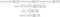Source

The model outputs the logits of two classes (class equivalent and class not equivalent). It can be done with just one logit followed by a sigmoid function since this is a binary class. Yet, it is quite common to use two logits. And it is not important which one to choose. Here is the output for 10 samples with two logit outputs each.

But the model is just randomly initialized. Next, we will restore it with the checkpoint from the remote directory. And we will train the model for 3 epochs again with an AdamW optimizer.

The AdamW optimizer’s we use will have a custom warmup period followed by gradual decay. The learning rate schedule will look like this: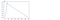Source

As a final task, we test the model with new samples. As a demonstration, we save and restore the model again.

TRecord

In real-life datasets, memory is not large enough to fit all samples. Instead, data is read from files when needed. But, for faster file reading and processing, we can save the data in the binary TRecord format designed for TF first. In the code below, we save samples as TFRecord files and creates datasets from them.

As a reference for advanced users, the code below has lower-level controls on the data loading.

This will be the code in creating the corresponding datasets:

TF Hub

TF Hub also supplies pre-trained TF Hub BERT models directly. Here is the code to create a BERT encoder, without the classification head. Then, we can add our own classifier head.

Or we can get a BERT classifier using classifier_model directly.

# Transformer for language translation

The diagram below is the general architecture for the Transformer. It is complex but important in deep learning. I do assume you have a basic understanding of the Transformer. If you cannot follow the discussion here, you should read this article first. In this example, we use a transformer to translate Portuguese to English.

Dataset preparation

First, we download the dataset file for translating Portuguese to English. We also prepare two tokenizers for the English and Portuguese samples respectively. The tokenizers have a limited vocabulary size. If a word is not in the vocabulary, the tokenizer will break it up into recognizable sub-words and tokenize each sub-word or word into an integer (token index). Here is the integer sequence for “Transformer is awesome.”.

Next, we add the start and end token of each sample.

Then, we create the datasets. But just as a demonstration, we drop samples with a token length over 40. In this example, many configurations will be scale down to speed up the training.

Word Embedding + Position Embedding

The Transformer uses learned embedding to convert a token index into a vector representation. To improve the model, the position of a word is also embedded (add) into the word embedding. To convert the scalar position pos into a 128-D vector, we use the sin and cosine function below for the even and odd elements in the vector respectively. (Note, the paper use a 512-D vector).

This is a visualization of the first 50 position values in a 512-D vector. The values are colorized according to the color bar on the right. As shown in the diagram, the position values in the early elements of the vector are recycled more frequently.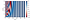Source

Padding

Padding is often applied to extend an input sequence to a specific-fixed length. For the input that should be ignored, the mask value is 1 otherwise 0. And this mask can pass to other layers in helping them to generate their output.

In addition, unlike a time sequence model, the Transformer makes all predictions concurrently during training. But in inference, it still predicts one word/sub-word at a time. During training, to avoid the attention module to peek into the future sequence, we create a mask to mask out this information.

Scaled Dot-Product Attention

Next, we create a Scaled Dot-Product Attention. The equation is

The diagram on the left is the model design.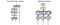Source

And here is the code.

Multi-head attention

Next, we implement multi-head attention using the scaled dot-product attention.

But, we don’t create 8 instances of scaled dot-product attention. Instead, the code below will reshape q, k, v properly such that all 8 heads can be processed by scaled dot-product attention as a single entity.

Position-wise Feed-Forward Network

Next, we implement an identical and shareable Position-wise Feed-Forward Network at each token location.

Encoder Layer

Each encoder layer will look like this:

But the code below has a dropout layer before both normalization layers.

Decoder layer

The decoder layer looks like the diagram below. It has 2 multi-head attention modules. The second one takes the output of the encoder to generate the keys K and values V.

Here is the code and it adds a dropout layer before the layer normalization layers again.

Encoder

Next, we stack up the Encoder layers to create an encoder.

We add the word embedding result with the position embedding together as the input to the encoder. The code also adds a dropout before the encoder.

Decoder

Now, we are ready to stack up decoder layers to create the decoder.

Transformer

Finally, we put the encoder and the decoder together to create a Transformer.

This encoder and the decoder have four stacked encoder layers and four decoder layers respectively. Each word is encoded as a 128-D vector by the Transformer encoder. The attention modules use 8 heads each. We use the dropout rate of 0.1.

Training

The training will use an Adam optimizer with a custom scheduler for the learning rates.

The remaining steps look similar to many other DL coding. So I will go through them quickly. Here are the loss function and performance metrics.

Next, we will create the Transformer, the CheckpointManager, and a function to create different masks for the encoder and the decoder according to the input.

This is the training step. The Transformer is not a sequential model. With the source sentence and the target sentence, we can predict the whole output sequence in one timestep. We just need to make sure that for each decoder’s token position, the attention module has the proper mask created such that it cannot see the future input sequence.

And the training loop.

Evaluation

After training, we use the Transformer to make translations. In inference, we predict one word/sub-word at a time only. We need the words predicted so far to predict the next word. So let’s trace it with the input sentence below.

`input: este é um problema que temos que resolverprediction: so this is a problem that we have to solve the global challenges`

The tokenizer converts the input sentence into a sequence of integer token indexes and then we add the start and end token to this sequence. This is the input to the Transformer encoder. The first input to the Transformer decoder will be the English start token. Now, we call the Transformer to make predictions. The transformer will predict a score for each word in the vocabulary used in the tokenizer. We will use the argmax to pick the next most likely word from these predictions. The returned value is the token index of the next word/sub-word.

Now we append this integer with the words we predicted so far. The predicted sequence contains the integer sequence for <s> and “so” now. In the next timestep, we feed this sequence as the new input to the decoder. The decoder will have two outputs, one for each decoder’s input token. We select the predictions for the last word and use argmax again. This time we pick the word “this”. We append this to the predicted sequence which is now <s>, “so”, “this”.

We use this sequence for the decoder in the next timestep. The decoder will have three outputs this time. Again, we use the predictions for the last position to choose our next word. We continue the iterations until the end token </s> is predicted. Here is the code for making a prediction for an input.

This is how we call it to translate a Portuguese sentence into English.

# Credits and References

All the source code is originated or modified from the TensorFlow tutorial.

Deep Learning